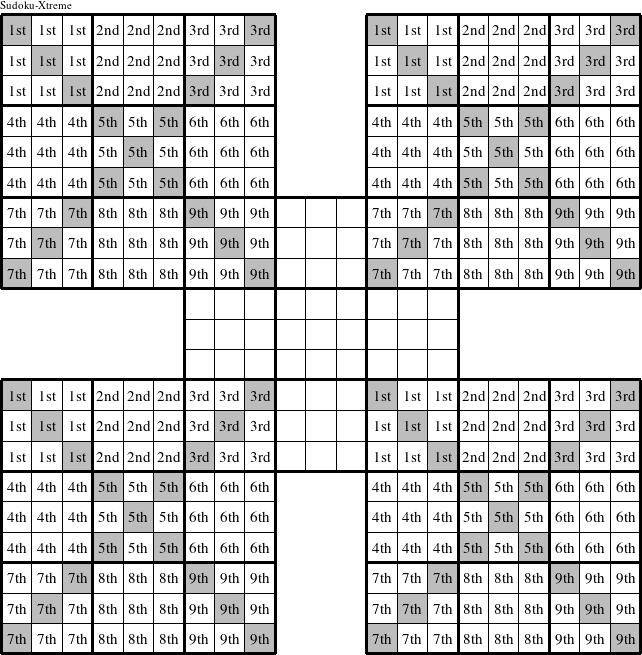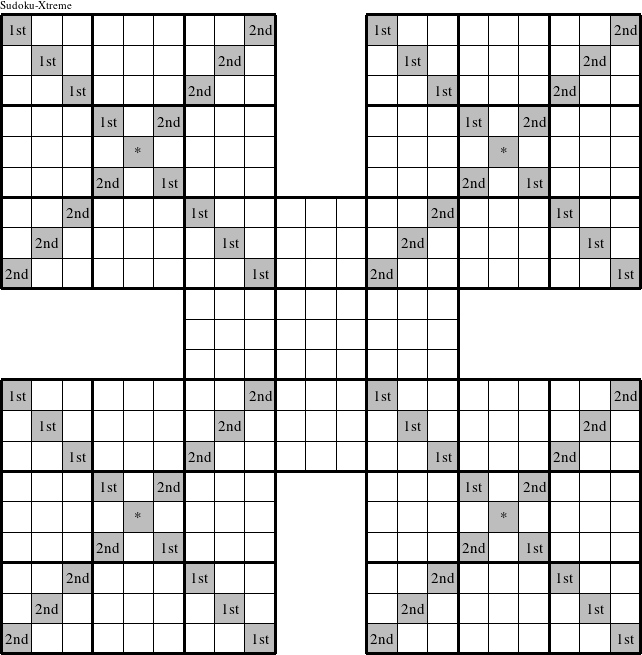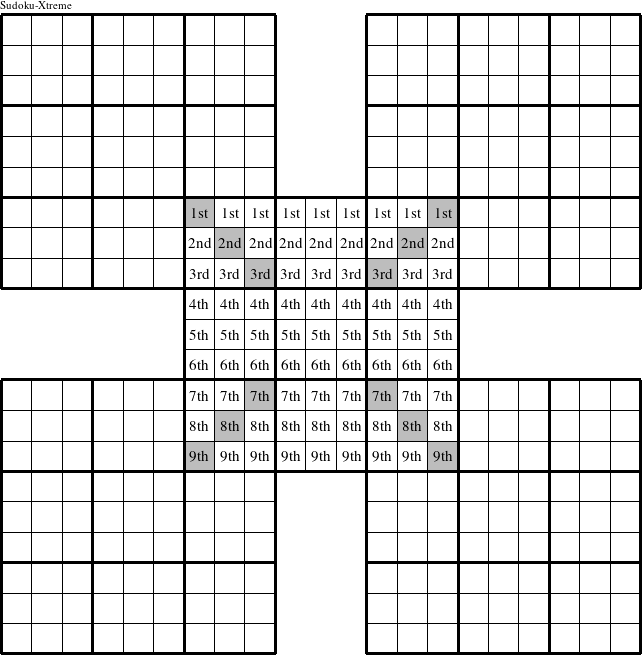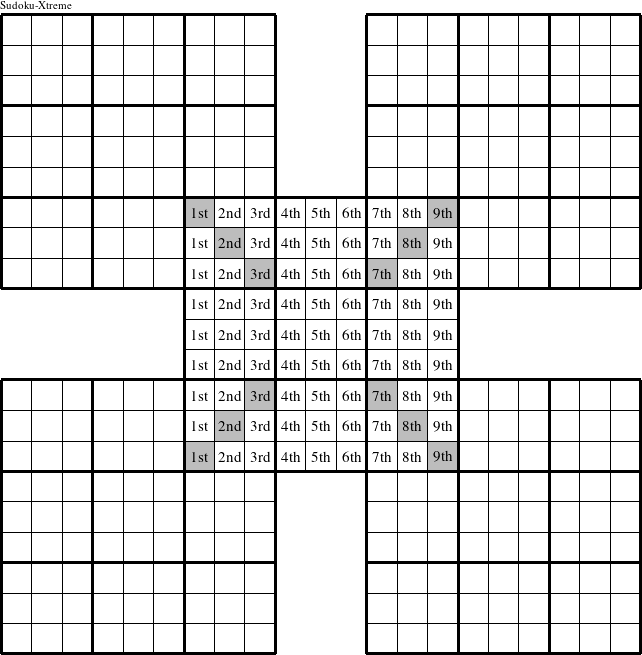#### Printable version

Detailed instructions for working a group puzzle of type 'Education-Xtreme'

This is a signature puzzle named 'Education-Xtreme'

The 9 elements in each group of this type of puzzle are: E, D, U, C, A, T, I, O, and N.

Every group in the puzzle must contain exactly this set of elements.

####In a Education-Xtreme puzzle, each row in the upper left, upper right, lower left, and lower right puzzles is a group numbered as shown in the figure above.

####In a Education-Xtreme puzzle, each column in the upper left, upper right, lower left, and lower right puzzles is a group numbered as shown in the figure above.

####In a Education-Xtreme puzzle, each 3x3 square in the upper left, upper right, lower left, and lower right puzzles is a group numbered as shown in the figure above.

####In a Education-Xtreme puzzle, each diagonal in the upper left, upper right, lower left, and lower right puzzles is a group numbered as shown in the figure above.

####In a Education-Xtreme puzzle, each row in the center puzzle is a group numbered as shown in the figure above.

####In a Education-Xtreme puzzle, each column in the center puzzle is a group numbered as shown in the figure above.

####In a Education-Xtreme puzzle, each 3x3 square in the center puzzle is a group numbered as shown in the figure above.